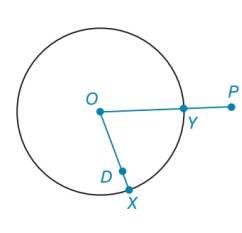Chapter 6.2, Problem 46E### Elementary Geometry for College St...

6th Edition
Daniel C. Alexander + 1 other
ISBN: 9781285195698

#### Solutions

Chapter
Section### Elementary Geometry for College St...

6th Edition
Daniel C. Alexander + 1 other
ISBN: 9781285195698
Textbook Problem
1 views

# In Exercises 39 to 47 , provide a paragraph proof. Be sure to provide a drawing, Given, and Prove where needed. Given: ⊙ O with P in its exterior; O - Y - P Prove: O P > O YTo determine

To prove:

The statement OP>OY.

Explanation

Calculation:

Given, a circle centered at O with the point P in its exterior.

Consider the given figure,

To prove OP>OY, draw a circle with radius OP as shown in the figure.

### Still sussing out bartleby?

Check out a sample textbook solution.

See a sample solution

#### The Solution to Your Study Problems

Bartleby provides explanations to thousands of textbook problems written by our experts, many with advanced degrees!

Get Started

#### Simplify: 728

Elementary Technical Mathematics

#### Evaluate the integral. 23. 01(1+x)8dx

Single Variable Calculus: Early Transcendentals

#### The center of 4x2 + 8x − 3y2 + 12y + 4 = 0 is (1, −2) (−1, 2) (2, −1) (−2, 1)

Study Guide for Stewart's Single Variable Calculus: Early Transcendentals, 8th